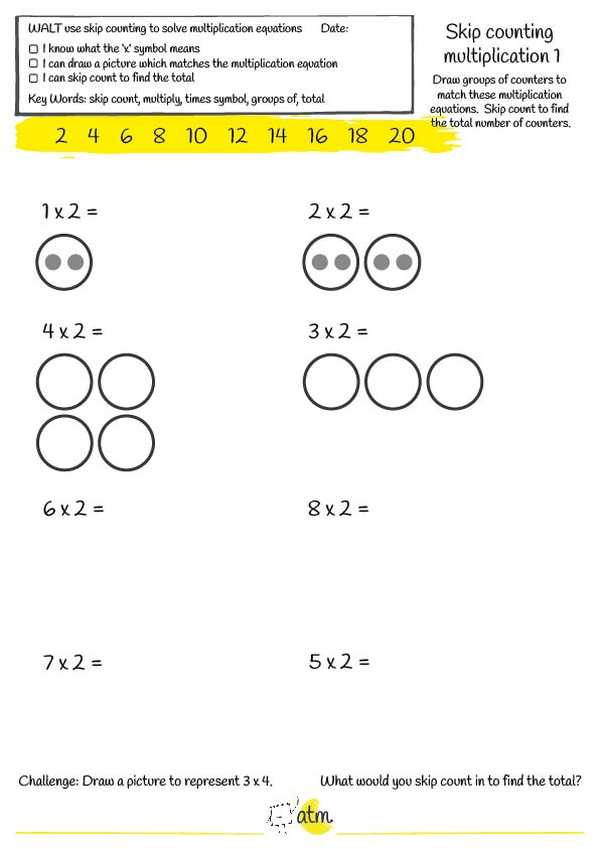#### Categories

Teaching/Maths/Skip Counting Multiplication

# Skip Counting Multiplication#### Description

2 pages, pdf

A worksheet stepping students through solving 2x multiplication problems using materials.

• Maths

#### Details

So you've laid the groundwork by introducing multiplication with materials, your students have done lots of practice and get the concept - now, you just need a worksheet to consolidate what that mysterious 'x' symbol means...ta da!

This download includes two worksheets with the Learning Intention WALT use skip counting to solve multiplication equations.

The first worksheet includes visuals showing groups of two to help students make connections between their use of materials and the written multiplication equation. The second worksheet provides a numberline to support students with skip counting in 2's and checks students' understanding of the multiplication symbol with word problems and challenge questions.

;